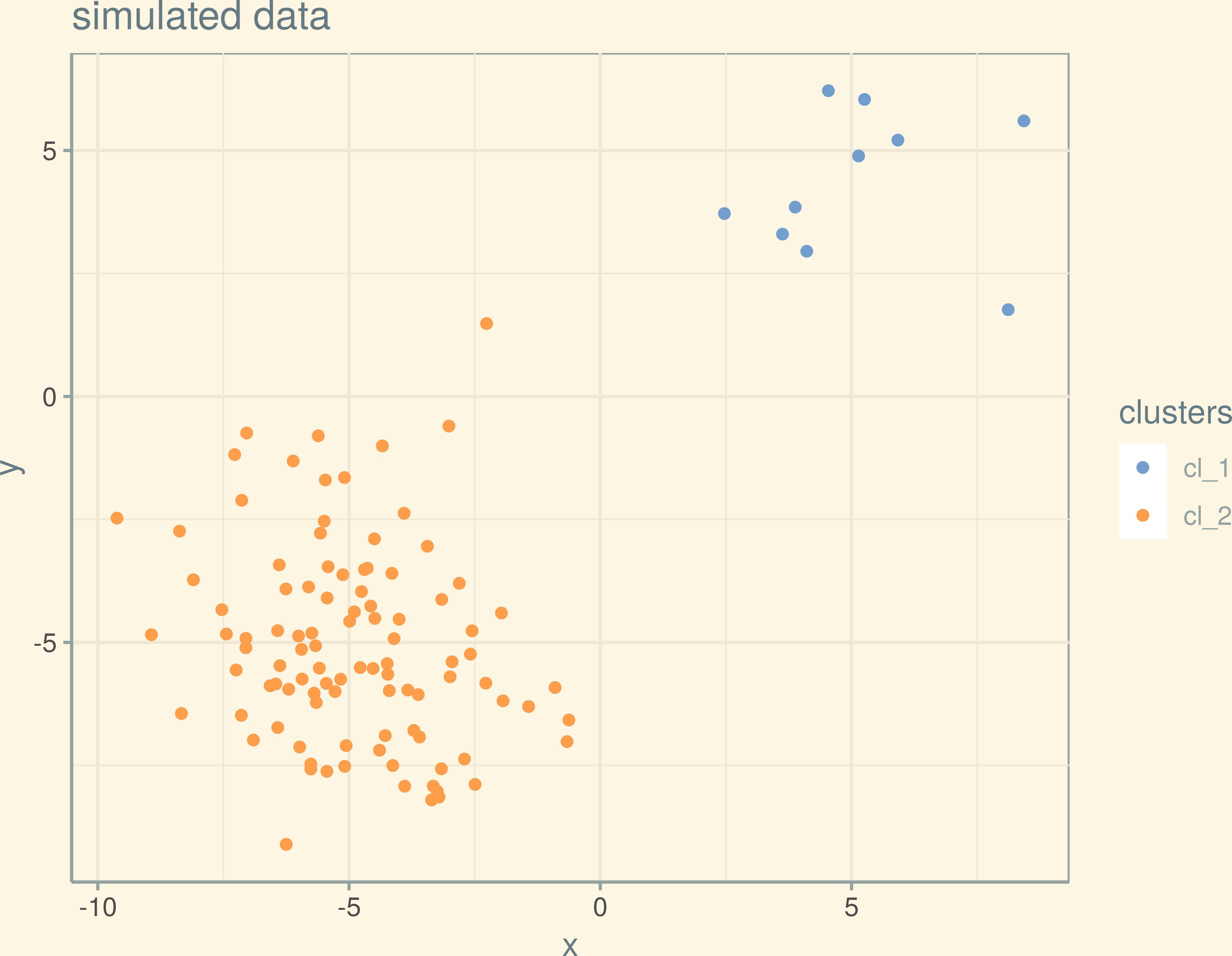# Contents

LAST UPDATE AT

``    "Tue Jun  5 15:47:12 2018"``

# 1 Packages and data import

We first set global chunk options and load the necessary packages and the data.

``````library("scran")
library("rmarkdown")
library("BiocStyle")
library("magrittr")
library("stringr")
library("ggthemes")
library("scales")
library("ggbeeswarm")
library("broom")
library("tidyverse")
library("ggrepel")
library("DESeq2")

if (!"patchwork" %in% rownames(installed.packages())){
devtools::install_github("thomasp85/patchwork")
}
library(patchwork)

data_dir <- file.path("../../Teaching/EMBL-Teaching/stat_methods_bioinf/data")

theme_set(theme_solarized(base_size = 18))

glog2 <- function(x) ((asinh(x) - log(2))/log(2))``````

# 2 Tools list for sc RNA–Seq

## 2.1 Alignment and quantification:

• “Conquer” workflow by Charlotte Soneson / Mark Robinson:

http://imlspenticton.uzh.ch:3838/conquer/

https://github.com/markrobinsonuzh/conquer

## 2.2 Preprocessing and QC

• Aaron Lun & Co preprocesing & QC workflow:

http://bioconductor.org/packages/release/workflows/html/simpleSingleCell.html

## 2.4 High level workflow

• Cave: uses zero inflated model [Perraudeau, Fanny, Davide Risso, Kelly Street, Elizabeth Purdom, and Sandrine Dudoit. 2017. “Bioconductor Workflow for Single-Cell RNA Sequencing: Normalization, Dimensionality Reduction, Clustering, and Lineage Inference]https://doi.org/10.12688/f1000research.12122.1

## 2.6 Dimensionlity reduction + graph building for sc Data

Single cell data is often used to infer (developmental) hierarchies of single cells. For this, a three step approach has emerged:

1. Dimenionality reduction

2. (optional) Clustering

3. Graph fitting

sincell is a Bioconductor package wrapping a couple of these techniques, typical examples includes monocle. Before one uses any of these algorithms, it is always a good idea to try to obtain robust clusterings via packages like clusterExperiment, as dimensionality reduction step can be misleading.

In fact, dimensionality reduction methods can result in trajectory–like patterns for random data. . This is often related to a certain covariance structure of the data. For an interesting example of an influential PCA misinterpretation in genetics see Novembre and Stephens (2008) Traditionally, wave-like patterns in PC maps have been interpreted as migration events. However, as they show, these patterns arise naturally as soon as genetic similarity decays with distance.

## 2.7 t–SNE maybe dangerous

### 2.7.1 Example: 2 clusters of different sizes

``````library("Rtsne")
run_tsne <- function(X, perplexity = 20, pca = FALSE, max_iter = 5000,
verbose = FALSE, is_distance = TRUE, seed=123L, ...){

set.seed(seed)

tX <- Rtsne(X, perplexity = perplexity, pca = pca, max_iter = max_iter,
verbose = verbose, is_distance = is_distance)\$Y

if(class(X) == "dist"){
labs <- labels(X)
} else {
labs <- rownames(X)
}

# browser()

colnames(tX) <- c("tSNE_dimension_1", "tSNE_dimension_2")
cell_id = labs,
.before = "tSNE_dimension_1")
tX

}``````
``````library("ggthemes")
set.seed(123)
x <- c(rnorm(10, mean = 5, sd = 2), rnorm(100, mean = -5, sd = 2))
y <- c(rnorm(10, mean = 5, sd = 2), rnorm(100, mean = -5, sd = 2))
clusters <- c(rep("cl_1", 10), rep("cl_2", 100))

dat_sim <- tibble(x, y, clusters)

org_plot <- ggplot(aes(x, y, color = clusters), data = dat_sim) +
geom_point() +
ggtitle("simulated data")

org_plot +
theme_solarized() +
scale_color_tableau("tableau10medium")``````• two normal clusters with different sizes, no within cluster structure

### 2.7.2 tSNEplot, perplexity = 5

``````X <- as.matrix(dat_sim[, 1:2])
rownames(X) <- dat_sim\$clusters

tsne <- run_tsne(X, perplexity = 5,
pca = FALSE, max_iter = 5000,
verbose = FALSE, is_distance = FALSE, seed = 123)

tsne_plot <- ggplot(tsne, aes(x = tSNE_dimension_1,
tSNE_dimension_2,
color = clusters)) +
geom_point(size = 3) +
ggtitle("t-SNE of sim data, perplexity = 5") +
coord_equal()
tsne_plot +
theme_solarized() +
scale_color_tableau("tableau10medium")``````